﻿ 单边直圆柔性铰链的柔度计算与分析 Compliance Calculation and Analysis of Half Right Circular Flexure Hinge

International Journal of Mechanics Research
Vol. 08  No. 02 ( 2019 ), Article ID: 30936 , 9 pages
10.12677/IJM.2019.82018

Compliance Calculation and Analysis of Half Right Circular Flexure Hinge

Yuemin Yu, Yuanzhu Ding, Liyan Yu, Fangfang Gai

College of Science, Heilongjiang University of Science & Technology, Harbin HeilongjiangReceived: Jun. 4th, 2019; accepted: Jun. 18th, 2019; published: Jun. 25th, 2019ABSTRACT

The compliance calculation formula of half right circular flexure hinge was derived on the Castigliano’s theorem. The influence of geometric parameters on compliance is studied. The correctness of the formula was verified by adopting the finite element simulation software. The results show that the relative errors of the half right circular flexure hinge are all less than 10%; the theoretical analysis is in agreement with the simulation results, which verifies the correctness of the proposed formula. These results will play a key theoretical basis for the structural design of half right circular flexure hinge in practical applications.

Keywords:Half Right Circular Flexure Hinge, Compliance, Castigliano’s Theorem, Finite ElementCopyright © 2019 by author(s) and Hans Publishers Inc.1. 引言

2. 单边直圆柔性铰链柔度模型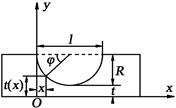Figure 1. Half right circular flexure hinge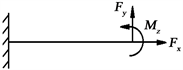Figure 2. Loads of half right circular flexure hinge

$\Delta =\left[\begin{array}{c}{\Delta }_{x}\\ {\Delta }_{y}\\ {\theta }_{z}\end{array}\right]=\left[\begin{array}{ccc}{C}_{11}& 0& 0\\ 0& {C}_{22}& {C}_{23}\\ 0& {C}_{32}& {C}_{33}\end{array}\right]\left[\begin{array}{c}{F}_{x}\\ {F}_{y}\\ {M}_{z}\end{array}\right]=CF$ (1)

${V}_{\epsilon }={\int }_{0}^{l}\frac{{F}_{x}^{2}\left(x\right)}{2EA\left(x\right)}\text{d}x+{\int }_{0}^{l}\frac{{\left[{M}_{z}+{F}_{y}\left(l-x\right)\right]}^{2}}{2EI\left(x\right)}\text{d}x$ (2)

3. 单边直圆柔性铰链柔度计算

$t\left(x\right)=R+t-R\mathrm{sin}\phi$ , $\phi \in \left(0,\text{π}\right)$ (3)

$x=R-R\mathrm{cos}\phi$ , $\text{d}x=R\mathrm{sin}\phi \text{d}\phi$ (4)

$A\left(x\right)=wt\left(x\right)$ (5)

$I\left(x\right)=\frac{wt{\left(x\right)}^{3}}{12}$ (6)

$\Delta x={C}_{11}{F}_{x}=\frac{\partial {V}_{\epsilon }}{\partial {F}_{x}}={\int }_{0}^{l}\frac{{F}_{x}}{EA\left(x\right)}\text{d}x={\int }_{0}^{\text{π}}\frac{{F}_{x}R\mathrm{sin}\phi }{Ew\left(R+t-R\mathrm{sin}\phi \right)}\text{d}\phi$ (7)

${C}_{11}=\frac{2N}{Ew\sqrt{{N}^{2}-1}}\left(\mathrm{arctan}\frac{N-1}{\sqrt{{N}^{2}-1}}+\mathrm{arctan}\frac{1}{\sqrt{{N}^{2}-1}}+\mathrm{arctan}\frac{N+1}{\sqrt{{N}^{2}-1}}\right)-\text{π}$ (8)

$\begin{array}{c}\Delta y={C}_{22}{F}_{y}+{C}_{23}{M}_{z}=\frac{\partial {V}_{\epsilon }}{\partial {F}_{y}}={\int }_{0}^{l}\frac{\left[{F}_{y}\left(l-x\right)+{M}_{z}\right]\left(l-x\right)}{EI\left(x\right)}\text{d}x\\ =\frac{12}{Ew}\left[{\int }_{0}^{\text{π}}\frac{{F}_{y}{\left(R+R\mathrm{cos}\phi \right)}^{2}R\mathrm{sin}\phi }{{\left(R+t-R\mathrm{sin}\phi \right)}^{3}}\text{d}\phi +{\int }_{0}^{\text{π}}\frac{{M}_{z}\left(R+R\mathrm{cos}\phi \right)R\mathrm{sin}\phi }{{\left(R+t-R\mathrm{sin}\phi \right)}^{3}}\text{d}\phi \right]\end{array}$ (9)

$\begin{array}{c}{C}_{22}=\frac{6}{Ew}\left\{\frac{1}{{\left({N}^{2}-1\right)}^{\frac{9}{2}}}\left[2{N}^{3}\left({N}^{4}-2{N}^{2}+1\right)\left(2{N}^{2}-5\right)×\left(\mathrm{arctan}\frac{N-1}{\sqrt{{N}^{2}-1}}+\mathrm{arctan}\frac{1}{\sqrt{{N}^{2}-1}}\right)\\ \text{\hspace{0.17em}}\text{\hspace{0.17em}}-{\left({N}^{2}-1\right)}^{\frac{5}{2}}\left(\text{π}{N}^{4}+2{N}^{3}-2\text{π}{N}^{2}-N-\text{π}-4\right)\right]\\ \text{\hspace{0.17em}}\text{\hspace{0.17em}}+\frac{1}{{\left({N}^{2}-1\right)}^{\frac{5}{2}}}\left[\sqrt{{N}^{2}-1}\left(-\text{π}{N}^{4}-2{N}^{3}+2\text{π}{N}^{2}+3N-\text{π}+4\right)+\mathrm{arctan}\frac{N+1}{\sqrt{{N}^{2}-1}}\left(5{N}^{5}-10{N}^{3}\right)\right]\right\}\end{array}$ (10)

$\begin{array}{l}{C}_{23}=\frac{12}{Ewl}\left\{\frac{1}{N{\left({N}^{2}-1\right)}^{\frac{5}{2}}}\left[6{N}^{2}\left(\mathrm{arctan}\frac{N-1}{\sqrt{{N}^{2}-1}}+\mathrm{arctan}\frac{1}{\sqrt{{N}^{2}-1}}\right)+\sqrt{{N}^{2}-1}\left(3{N}^{2}+2N+2\right)\right]\\ \text{\hspace{0.17em}}\text{\hspace{0.17em}}\text{\hspace{0.17em}}\text{\hspace{0.17em}}\text{\hspace{0.17em}}\text{\hspace{0.17em}}\text{\hspace{0.17em}}\text{\hspace{0.17em}}+\frac{1}{{\left({N}^{2}-1\right)}^{\frac{5}{2}}}\left(N\sqrt{{N}^{2}-1}-2\sqrt{{N}^{2}-1}+6\mathrm{arctan}\frac{N+1}{\sqrt{{N}^{2}-1}}\right)\right\}\end{array}$ (11)

$\begin{array}{c}{\theta }_{z}={C}_{33}{M}_{z}+{C}_{32}{F}_{y}=\frac{\partial {V}_{\epsilon }}{\partial {M}_{z}}={\int }_{0}^{l}\frac{{M}_{z}+{F}_{y}\left(l-x\right)}{EI\left(x\right)}\text{d}x\\ =\frac{12}{Ew}\left[{\int }_{0}^{\text{π}}\frac{{M}_{z}R\mathrm{sin}\phi }{{\left(R+t-R\mathrm{sin}\phi \right)}^{3}}\text{d}\phi +{\int }_{0}^{\text{π}}\frac{{F}_{y}\left(R+R\mathrm{cos}\phi \right)R\mathrm{sin}\phi }{{\left(R+t-R\mathrm{sin}\phi \right)}^{3}}\text{d}\phi \right]\end{array}$ (12)

$\begin{array}{c}{C}_{33}=\frac{24}{Ew{l}^{2}}\left\{\frac{1}{N{\left({N}^{2}-1\right)}^{\frac{7}{2}}}\left[6{N}^{2}\left(\mathrm{arctan}\frac{N-1}{\sqrt{{N}^{2}-1}}+\mathrm{arctan}\frac{1}{\sqrt{{N}^{2}-1}}\right)+\left(2{N}^{2}+1\right){\left({N}^{2}-1\right)}^{\frac{3}{2}}\right]\\ \text{\hspace{0.17em}}\text{\hspace{0.17em}}+\frac{1}{N{\left({N}^{2}-1\right)}^{\frac{5}{2}}}\left[\sqrt{{N}^{2}-1}\left(2{N}^{2}+1\right)+6{N}^{2}\mathrm{arctan}\frac{N+1}{\sqrt{{N}^{2}-1}}\right]\right\}\end{array}$ (13)

$\begin{array}{l}{C}_{32}={C}_{23}\\ =\frac{12}{Ewl}\left\{\frac{1}{N{\left({N}^{2}-1\right)}^{\frac{5}{2}}}\left[6{N}^{2}\left(\mathrm{arctan}\frac{N-1}{\sqrt{{N}^{2}-1}}+\mathrm{arctan}\frac{1}{\sqrt{{N}^{2}-1}}\right)+\sqrt{{N}^{2}-1}\left(3{N}^{2}+2N+2\right)\right]\\ \text{\hspace{0.17em}}\text{\hspace{0.17em}}+\frac{1}{{\left({N}^{2}-1\right)}^{\frac{5}{2}}}\left(N\sqrt{{N}^{2}-1}-2\sqrt{{N}^{2}-1}+6\mathrm{arctan}\frac{N+1}{\sqrt{{N}^{2}-1}}\right)\right\}\end{array}$ (14)

4. 柔度的实例计算及有限元验证

4.1. 结构参数对柔度性能影响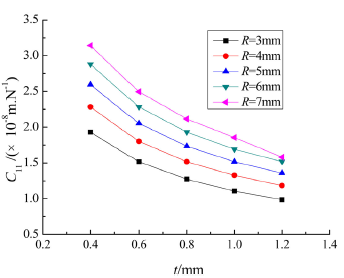Figure 3. The relation between C11 and t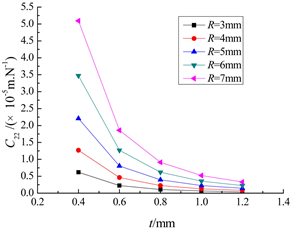Figure 4. The relation between C22 and t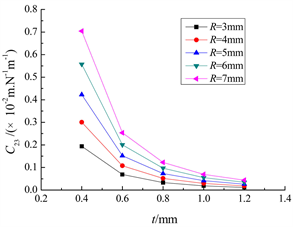Figure 5. The relation between C23 and t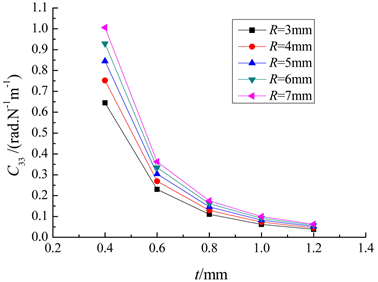Figure 6. The relation between C33 and t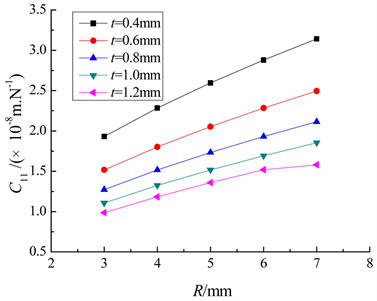Figure 7. The relation between C11 and R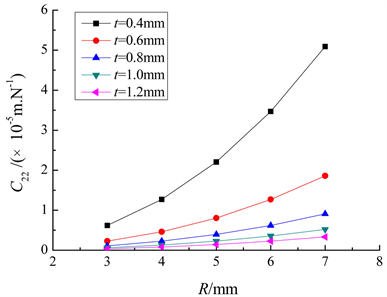Figure 8. The relation between the C22 and R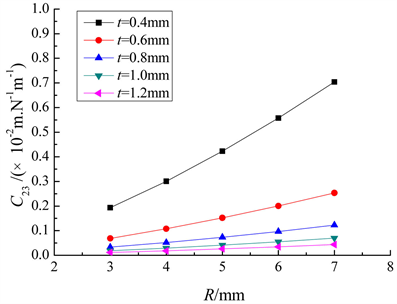Figure 9. The relation between C23 and R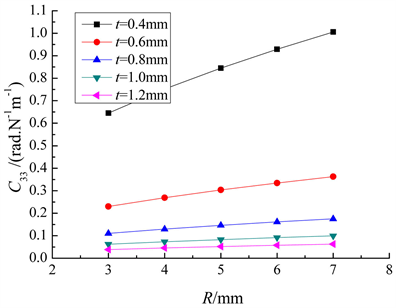Figure 10. The relation between C33 and R

4.2. 有限元分析Table 1. Material parameters of half right circular flexure hingeTable 2. The results contrast between compliance computation and finite element analysis

5. 结论

Compliance Calculation and Analysis of Half Right Circular Flexure Hinge[J]. 力学研究, 2019, 08(02): 156-164. https://doi.org/10.12677/IJM.2019.82018

1. 1. 卢清华, 黄世乾. 一种3自由度精密微定位平台的设计与分析[J]. 机械设计与研究, 2014, 30(5): 56-59.

2. 2. 李春霞, 赖磊捷, 朱利民. 三自由度θX θY Z柔性机构的柔度分析与计算工[J]. 工程力学, 2014, 31(8): 204-222.

3. 3. 崔玉国, 王子宾, 马剑强, 等. 四自由度压电微夹钳的设计[J]. 机械工程学报, 2017, 53(23): 165-173.

4. 4. Paros, J. (1965) How to Design Flexure Hinges. Journal of Mechanical Design, 37, 151-156.

5. 5. Smith, T.S. and Badami, V.G. (1997) Elliptical Flexure Hinge. Review of Scientific Instruments, 68, 1474-1483. https://doi.org/10.1063/1.1147635

6. 6. Lobontiu, N. and Paine, J.S. (2001) Corner-Filleted Flexure Hinges. Journal of Mechanical Design, 123, 346-352. https://doi.org/10.1115/1.1372190

7. 7. Lobontiu, N. (2002) Design of Circular Cross-Section Corner-Filleted Flexure Hinges for Three-Dimensional Compliant. Journal of Mechanical Design, 124, 479-484. https://doi.org/10.1115/1.1480022

8. 8. 陈贵敏, 刘小院, 贾建援. 椭圆柔性铰链的柔度计算[J]. 机械工程学报, 2006, 23(s1): 111-115.

9. 9. 杨春辉. 倒圆角直梁型柔性球铰柔度计算与分析[J]. 机床与液压, 2013(3): 55-58.

10. 10. 杨春辉, 刘平安. 圆弧形柔性球铰柔度设计计算[J]. 工程设计学报, 2014, 21(4): 389-404.

11. 11. 邱丽芳, 南铁玲, 翁海珊. 柔性铰链运动性能多目标优化设计[J]. 工程科学学报, 2008, 30(2): 189-192.

12. 12. 姚建涛, 李立建, 杨维, 等. 直圆柔性球铰柔度矩阵的解析计算[J]. 光学精密工程, 2014, 2(7): 1857-1863.

13. 13. 陈应舒, 朱淳逸. 曲梁柔性铰链性能分析及应用机构设计[J]. 机械设计与制造, 2018(11): 183-185.

14. 14. 张志杰, 袁怡宝, 于汶. 单边椭圆柔性铰链的计算与性能分析[J]. 机械设计与研究, 2007, 23(1): 50-53.

15. 15. 张志杰, 袁怡宝. 单边导角形柔性铰链的计算与性能分析[J]. 光学精密工程, 2007, 15(3): 384-389.